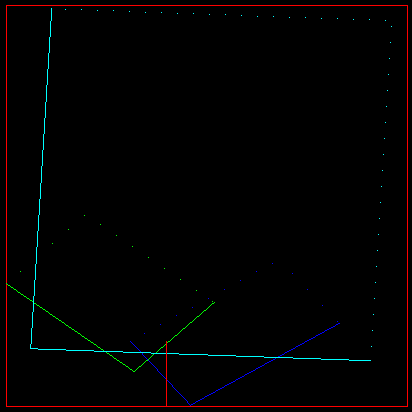# QB CULT MAGAZINEVol. 3 Iss. 2 - November 2003

## Fractals with QB

By Jark <http://mandelbrot.dazibao.free.fr>

### Fractals attraction

Fractals are attractive for three reasons:

• They break most of the conventional mathematical rules
• They are an endless subject for programmers
• They are a nice and easy way to produce impressive graphics

This article will give you the essential notions about fractals, and will also put you on the track for great graphics programming, with professional results even with Quick-Basic.

### Fractal dimension

Geometry was based, until the late seventies, on integer dimensions: objects were always one, two or three dimensional entities, placed in one, two or three dimensions spaces.

Dimensions have two roles:

• locate the objects in the space
• measure the sizes and distances

Then came fractals... And dimensions would never be the same!

Just consider the flock of Von Koch, built on iterative divisions of a triangle:This object has an infinite length: no dimension trick allows telling what size it is... and the best thing you can tell about a point is "We are sure this point is on the line", or "We are sure this point is not on the line", provided you have computed a couple of iterations, and provided your computer did not run out of memory while doing this. You just cannot say: "I got the point which lies at distance D on the line, starting from the origin".

So mathematicians had to generalise the dimension notion, to allow non-integer cases: the Flock of Koch, or example, is a 1.2619 dimension object.

### Programming fractals

Fractals like the flock of Von Koch, or like the Triangle of Sierpinsky, can be approximated in 2D with simple programs.The basis is always the same:

1. Identify and define the elementary object: a line, a triangle, a circle...
2. Program the elementary operation that splits the basis object to next rank objects
3. Store the result in an array
4. Plot the result

You can study the example of the Apollonia fractal, built as follows:

• The initial pattern is made of three circles, tangent two by two
• These three circles generate one and one only circle, called the Soddy Circle, which is tangent to the three original ones
• Repeat and repeat and repeat...Solving the Soddy circle is quite easy:

Given three circles (xi,yi,Ri), the Soddy circle (x,y,R) complies with the three equations (i ranging from 1 to 3):

(x-xi)^2 + (y-yi)^2 = (R + Ri)^2

Developing these three equations and subtracting them two by two provides a nice linear system in x, y, and R. So we can have x and y as functions of R, and plug that into one of the three original equations. That will give a 2nd degree polynomial equation in R, solvable via the classical discriminant method.

Download and tweak to your taste Apollo12.bas, and play with this easy Screen 12 fractal code. It can plot:

• the flock of Von Koch
• the triangle of Sierpinsky
• the Apollonia fractal
• the Apollonia gasket

Of course, you can think about making the same in 3D: but since you are a QB progger, you must remember that the number of objects grows extremely rapidly! Take a look at that not-so-simple Sierpinsky Sponge, made of 8000 raytraced elementary cubes.### Iterative Functions Systems

The pictures above are still really geometric... You may have heard about Barnsley's fern, or about IFS. This fractal object looks really natural, still, it is based on a simple mathematical trick. Imagine a photocopier that would not only reduce or enlarge the original picture, but also twist the global layout, thus transforming a rectangle into a parallelogram. This is called an "affin transformation":

• xf = a1 * x + b1 * y + c1
• yf = a2 * x + b2 * y + c2

We can represent a transformation by the corresponding parallelogram, and define, let's say 4 transformations.Let's pick a point at random in the original square. This point will be copied 4 times by our strange copier. These 4 points will be copied 4 times each, and so on, and so on...

In the end, you will get something like this fern:Well, I admit I went too fast: if you check this program, you will see that the frames themselves are plotted, not the point, to save computing time. But the principle remains the same...

The most amazing about this random approach is that you can plot the Sierpinsky's triangle with the same rational: just check this flowers generator and see by yourself that the random copier principle can make the same pic as above.

The Mandelbrot Dazibao... Think Global, Make Symp'All! ®